# Electrical Engineering test questions for exams and entrance - 7

## Electrical Engineering test questions for exams and entrance - 7

(1) By an 8085 microprocessor, the instructions given below have been executed.

6010 LXI H, 8AY9 H
6013 MOV A, L
6016 DAA
6017 MOV H, A
6018 PCHL

The next instruction will be fetched from address

(A) 6019
(B) 6379
(C) 6259
(D) 6240

(2) Local oscillators in a BPSK signal detector, has a fixed phase error of 20° that deteriorates SNR at the output, by a factor of

(A) cos70°
(B) cos90°
(C) cos120°
(D) cos45°

(3) Rated load torque at rated speed is delivered by a dc cumulatively compounded motor and if series field is short-circuited then,

(A) The armature current will decrease and speed will increase
(B) The armature current will increase and speed will decrease
(C) The armature current and speed both will decrease.
(D) The armature current and speed both will increase

ANSWER: The armature current and speed both will increase

(4) In the circuit shown below it is desired to have a constant direct current i (t) through an ideal inductor L. The nature of the voltage source v (t) must be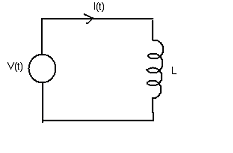(A) Linearly increasing voltage
(B) An ideal impulse
(C) An exponentially increasing voltage.
(D) Constant voltage

(5) Consider the circuit shown below. When viewed from the terminal AB this circuit can be reduced to an equivalent circuit at single voltage source in series with a single resistor with

the parameters as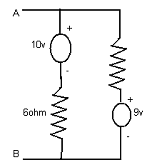(A) 10 volt source in series with 10ohm resistor
(B) 5 volt source in series with 10ohm resistor
(C) 15 volt source in series with 15ohm resistor
(D) 1 volt source in series with 2.4ohm resistor

ANSWER: 1 volt source in series with 2.4ohm resistor

(6) A feedback control system has characteristic equation as 2s4 + s³ + 3s² + 5s + 10 = 0. How many roots are present in the right half of s plane?

(A) 3
(B) 2
(C) 1
(D) 0

(7) For power measurement a two-wattmeter method is used. What should be the load pf angle for one of the wattmeter to show negative reading?

(A) Less than 60°
(B) Less than 45°
(C) Greater than 60°
(D) Greater than 45°

(8) When a sinusoidal current i (t) = 10 sin ωt is passed through a rectifier type ammeter, it indicates a current reading of 7.07 A. The waveform shown below is of current that is

passed through a rectifier type instrument. The true RMS current in the circuit is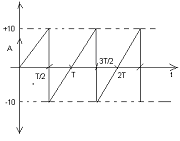(A) 7.07 A
(B) 3.20 A
(C) 1.26 A
(D) 5.87 A

(9) For the K map shown below the minimal product of sum function described is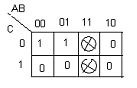(A) A’C’
(B) A + C
(C) AC
(D) A’ + C’

(10) Consider a 3-phase, 4-pole squirrel cage induction motor that has 36 stator and 28 rotor slots. The number of phases in the rotor is

(A) 7
(B) 10
(C) 3
(D) 5

(11) The torque speed characteristic of an electric motor with constant output power is in the form of

(A) Rectangular hyperbola
(C) Straight line through the origin
(D) Straight line parallel to the speed axis.

(12) In smooth cylindrical conductors in air at NTP disruptive corona begins, if electric field intensity at the conductor surface goes up to

(A) 21.1 Kv (peak)/cm
(B) 21.1 Kv (rms)/cm
(C) 21.1 Kv (average)/cm
(D) 21.1 Kv (rms)/m

(13) What is the reflection coefficient for the transmission line at point P?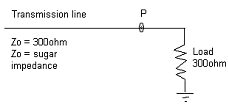(A) ∞
(B) -1
(C) 1
(D) 0

(14) At an angle of 30° with the vertical a radiowave is incident on a layer of ionosphere. What will be the maximum usable frequency if critical frequency is 1.2 MHz?

(A) 2.646 MHz
(B) 0.356 MHz
(C) 1.386 MHz
(D) 1.843 MHz

(15) The rotor circuit of a 6-pole 50 Hz induction motor makes 30 oscillations in one minute considering a center zero ammeter connected to a rotor circuit. The speed of the rotor is

(A) 990
(B) 1000
(C) 500
(D) 1500

(16) Internal resistance of a 100μA ammeter is 100 ohm. What should be the value of shunt resistance required in order to extend its range to measure 500μA?

(A) 22ohm
(B) 25ohm
(C) 20ohm
(D) 50ohm

(17) For the 2-phase servomotor X / R ratio is (where X is rotor reactance and R is rotor resistance)

(A) Less than or greater than that of a normal induction motor
(B) Greater than that of a normal induction motor
(C) Equal to that of a normal induction motor
(D) Less than that of a normal induction motor

ANSWER: Less than that of a normal induction motor

(18) Let firing angle in a 3-phase semi-converter be = 60°. The duration of conduction of each thyristor and diode would be respectively

(A) 120° and 60°
(B) 120° and 120°
(C) 60° and 120°
(D) 60° and 60°

(19) Consider the circuit shown below. This circuit is equivalent to a load of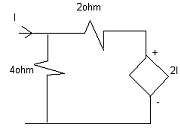(A) 1/4 ohms
(B) 3/2 ohms
(C) 8/3 ohms
(D) 3/8 ohms

(20) The admittance parameters of a two port network shown in the figure are Y11 = 8 mho, Y12 = Y21 = -6 mho, Y22 = 6 mho. What will be the respective values of YA, YB and YC in the units

of mho?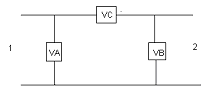(A) 2, 5 and 8
(B) 2, 0 and 6
(C) 2, 6 and 0
(D) 2, 1 and 4

(21) To give rated output of 1500rpm a 240V dc series motor takes 40 A current. Its resistance is 0.3 ohms. To obtain rated torque at 1000rpm, the value of resistance, which must be added,

is

(A) 5 ohms
(B) 2.3 ohms
(C) 1.9 ohms
(D) 6.9 ohms

(22) The starting address of an 8085 microprocessor based system that uses a 4K x 8 bit RAM is AA00 H. In this RAM the address of the last byte is

(A) BA00 H
(B) 1000 H
(C) B9FF H
(D) 0FFF H

(23) Consider a JFET whose gm is 1 mA/V and Cgs + Cgd + Cgss = 5 pF. Symbols have their usual meaning. Ft of the JFET is nearly equal to

(A) 1 MHz
(B) 10 MHz
(C) 20 MHz
(D) 30 MHz

For Questions 24 and 25 refer to the data given below:

X = 20nc and Y = 10nc are the two point charges separated from each other at a distance of 25 cm in free space.

(24) A point D is 15 cm away from point X and 20 cm away from point Y. The electric field at point D is

(A) 8.31 kV/m
(B) 9.2 kV/m
(C) 3.2 kV/m
(D) 4.5 kV/m

(25) The angle θ is

(A) 15.7°
(B) 9.8°
(C) 21.9°
(D) 11.2°